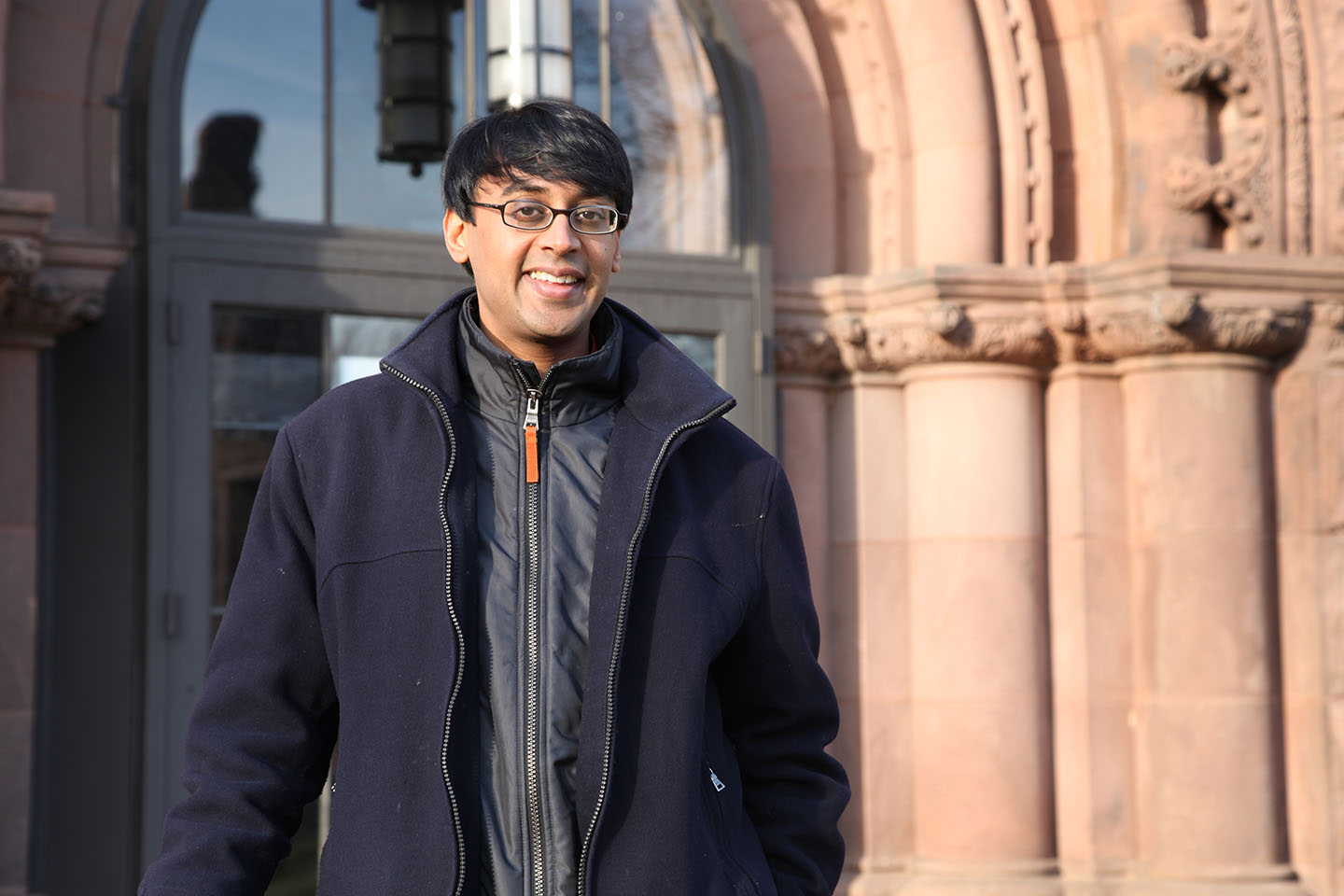## 2015 Mahler Lecturer: Professor Manjul BhargavaPhoto courtesy of Infosys Science Foundation

The Mahler lectures are a biennial activity organised by the Australian Mathematical Society, and supported by the Australian Mathematical Sciences Institute, in which a prominent mathematician tours Australian universities giving lectures at a variety of levels, including giving several public lectures.

In 2009 AMSI and AustMS partnered with the Clay Mathematical Institute, to combine the Mahler Lectures and the Clay Lectures into the Clay–Mahler Lecture Tour.

Biography

Bhargava is currently a Professor of Mathematics at Princeton University.

Bhargava’s thesis provided a reformulation of Gauss’s law for the composition of two binary quadratic forms. He showed that the orbits of the group SL(2, Z)3 on the tensor product of three copies of the standard integral representation correspond to quadratic rings (rings of rank 2 over Z) together with three ideal classes whose product is trivial. This recovers Gauss’s composition law in an original and computationally effective manner. He then studied orbits in more complicated integral representations, which correspond to cubic, quartic, and quintic rings, and counted the number of such rings with bounded discriminant.

Bhargava next turned to the study of representations with a polynomial ring of invariants. The simplest such representation is given by the action of PGL(2, Z) on the space of binary quartic forms. This has two independent invariants, which are related to the moduli of elliptic curves. Together with his student Arul Shankar, Bhargava used delicate estimates on the number of integral orbits of bounded height to bound the average rank of elliptic curves. Generalizing these methods to curves of higher genus, he recently showed that most hyperelliptic curves of genus at least two have no rational points.

Bhargava’s work is based both on a deep understanding of the representations of arithmetic groups and a unique blend of algebraic and analytic expertise.

Tour Program

Information to follow

Talk abstracts

Information to follow